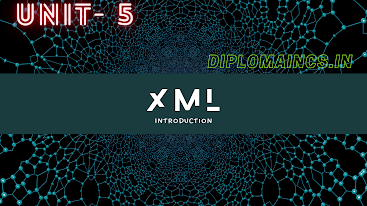## 2/25/20

### Previous year question of database management system 2019

Sub Code: - 1618403

2019(Even)
Time : 3Hrs
Semester  IV(New)
DBMS
Full Marks : 70
Pass marks : 28

Group A

Choose the most suitable answer from the following options: -   (1*20=20)

(i) Which is not the relational Algebra Operation

(a) Set Difference
(b) Cartesian product
(c) Rename
(d) Join

(ii) How is specialization denoted in ER diagram?

(a) Triangle labeled IS A
(b) Rectangle labeled IS A
(c) Rectangle labeled HAS A
(d) Triangle labeled HAS A

(iii) Which of the following is open source DBMS?

(a) MySql
(b) SQL server
(c) Microsoft Access
(d) Oracle

(iv) Which SQL keyword is used to remove the duplication of rows from the result set?

(a)DELETE
(b) SELECT
(c) DISTINCT
(d)  UNIQUE

(v) What is the highest level in a hierarchy of data organization

(a) Data record
(b) Data field
(c) Data base
(d) None of these

(vi) Which of the following is known as minimal super key?

(a) Primary key
(b) Foreign key
(c) Unique key
(d) Candidate key

(vii) Which command allow the users to change multiple data fields?

(a) Modify
(b) Look up
(c) Update
(d) Change

(viii) Which of the following languages is used to define the integrity constraint.

(a) DCL
(b) DML
(c) DDL
(d) All of these

(ix) In an ER diagram attributes are represented as:

(a) Rectangle
(b) Square
(c) Ellipse
(d) Triangle

(x) Architecture of data base can be viewed as

(a) Two levels
(b) Four levels
(c) Three-levels
(d) One-level

(xi) In relational model, relations are termed as

(a) Tuples
(b) Attributes
(c) Table
(d) Row

(xii) A Cartesian product in relational algebra in

(a) Unary Operator
(b) Binary operator
(c) Ternary operator
(d) None of these

(xiii) SQL stands for

(a) Structured Query Language
(b) Sequential Query Language
(c) Structured Question Language
(d) None of these

(xiv) The following are component of a data base except

(a) User data
(b) Meta data
(c) Reports
(d) Indexes

(xv) A set of possible data values is?

(a) Attribute
(b) Degree
(c) Turple
(d) Domain

(xvi) Which of the following data base objet does not physically exist?

(a) Base table
(b) Index
(c) View
(d) None of these

(xvii) Cross product is a

(a) Unary operator
(b) Ternary operator
(c) Binary operator
(d) None of the above

(xviii) In E-R diagram relationship is represented by

(a) Ellipse
(b) Dashed line
(c) Rectangle
(d) Diamond

(xix) To delete a particular column in a relation the command used is?

(a) UPDATE
(b) DROP
(c) ALTER
(d) DELETE

(xx) A table joined with itself is called

(a) Join
(b) Self join
(c) Quter join
(d) Equi join

Group:-"B"

Answer all Five Questions: -                         (5*4=20)

2. Distinguish between primary key and super keys.
OR
Explain about different types of users in DBMS?

3. What is view? Explain it.
OR
Draw the E-R diagram for hospital management.

4. Write short notes on nested queries.
OR
List and explain aggregate functions used in SQL with examples.

5. Write the structure of SQL SELECT statements
OR
What is transaction? Explain the properties of transaction.

6. Describe the characteristics of DBMS.
OR
List SQL grouping functions with examples.

Group:- "C"

Answer all Five Questions: -                         (5*6=30)

7. Consider the relation schemes given below:
STUDENT (student-id, name)
ENROLLED in (student-id, subject code)
SUBJECTS (subject code, Lectures)
Write relation algebra query for
i.                 Who teaches CP 1500 or CP 3020
ii.               Who teaches at least two different subjects
OR
Explain the roles of DBA? Describe 3 tier data base Architecture of DBMS?

8. Draw an E-R Diagram for following data:
A dept store operations in several cities. In a city there is one head quarters. A city may have several stores. Stores hold any amount of items. Customers can order any number of items.
OR
Consider the following relations and write queries in SQL:
Flights (fl no, from, to, distance, departs, arrives, price)
Aircrafts (a id, a name, cruising-range)
Certified (e id, a id)
Employees (e id, e name, salary)
i.                 Identify the e id of employees who make the highest salary
ii.               Print the names and salary of every non pilot

9. Why normalization is needed? Explain the process of normalization.
OR
Explain about Boyce Code Normal Form with an example.

10. Discuss in detail about SELECT, PROJECT and UNION with suitable SQL query
OR
What do you mean by composite attribute and derived attribute? Give example

11. Consider the relation R= {A, B, C, D, E ,F ,G ,H, I, J}
And set up FD’s = {AB -> C,  A -> DE, B -> F, F -> GH, D -> IJ} decompose R in 3NF.
OR
Consider the relation R (A, B, C) and set of FD’s F= { A-> BC, B- > C, A-> B, AB->C}
Compute the canonical cover of F.